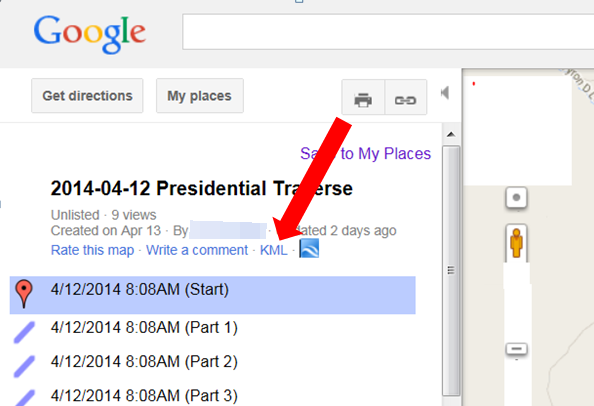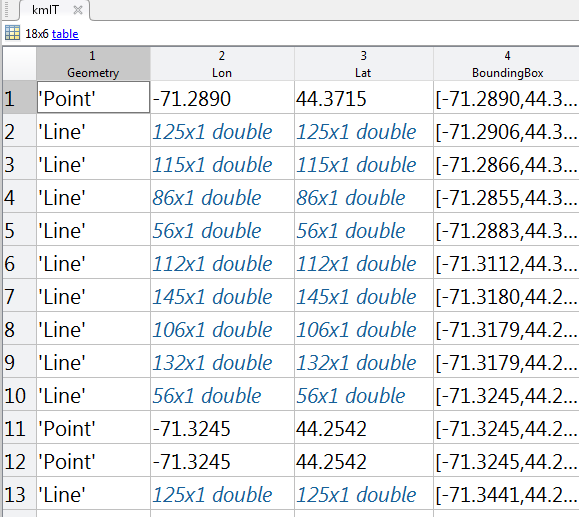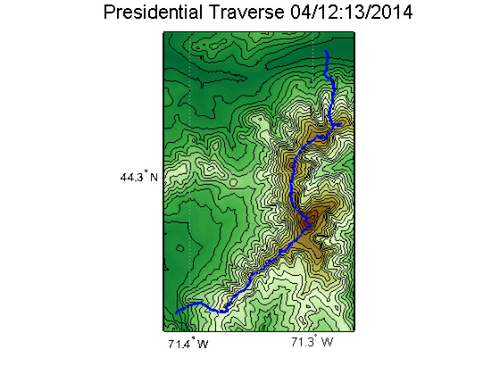# KML2STRUCT – Easily Import Your KML Files

Sean's pick this week is kml2struct by James Slegers.

Earlier this week, my friend sent me a Google Maps link containing our hiking tracks recorded with the GPS on his smart phone. From Google Maps you can download the data as a KML (Keyhole Markup Language) file.I wanted to plot it and experiment with the data in MATLAB. Once again, the File Exchange was there for me!

% Read the KML file into a struct:
kmlS = kml2struct('2014-04-12PresidentialTraverse.kml');

% Convert to table (new datatype in R2013b) to make manipulations easier
kmlT = struct2table(kmlS);

Now looking at the table, we can see the four important pieces:

• Geometry: What is it? A point, line, etc.
• Lon: Longitude coordinate of tracks
• Lat: Latitude coordinate of tracks
• Bounding Box: Bounding box if we want to draw it on a mapFirst, I'll get the bounding box of the whole trip. To do this, we'll stack each segment's bounding box into the third dimension and then pick the min and the max:

boxes = kmlT.BoundingBox; % Extract Bounding box from table
boxes3d = cat(3,boxes{:}); % Stack along third dimension
bbox = [min(boxes3d(1,:,:),[],3); max(boxes3d(2,:,:),[],3)].'; % Min and max along third dimension give limits
latlim = bbox(2,:)+[-0.01 0.01]; % Buffer them
lonlim = bbox(1,:)+[-0.01 0.01];

Next, I only want to work with the lines, i.e. the actual tracks. The points represent termini, which I don't need right now. Using the new categorical data type and logical indexing, we can extract the latitude and longitude from the table.

% Make Geometry categorical
kmlT.Geometry = categorical(kmlT.Geometry);

% Extract the latitude and longitude for the lines
latlon = kmlT{kmlT.Geometry=='Line', {'Lat','Lon'}};

I'll get the elevation data from NASA using the Web Map Service in the Mapping Toolbox.

nasaLayers = wmsfind('nasa*elev', 'SearchField', 'serverurl');
ned = refine(nasaLayers, 'usgs_ned');
[Z, refmatZ] = wmsread(ned, 'Latlim', latlim, 'Lonlim', lonlim);
Z = double(Z);

And finally, plot a contour map with the tracks overlaid on it.

figure
ax = usamap(latlim, lonlim);
geoshow(Z, refmatZ, 'DisplayType', 'texturemap')
contourm(Z, refmatZ, 20, 'Color', 'k')
demcmap(Z)
title('Presidential Traverse 04/12:13/2014','FontSize',16)

for ii = 1:length(latlon)
geoshow(latlon{ii,:}, 'LineWidth', 2)
endHave you ever recorded a trip and then tried to analyze it in MATLAB? The tasks above would be more straight-forward if you had access to the original GPX files. These typically come with the elevation data and time stamps allowing you to get even more statistics with more accuracy.

Give it a try and let us know what you think here or leave a comment for James.

Published with MATLAB® R2014a

|